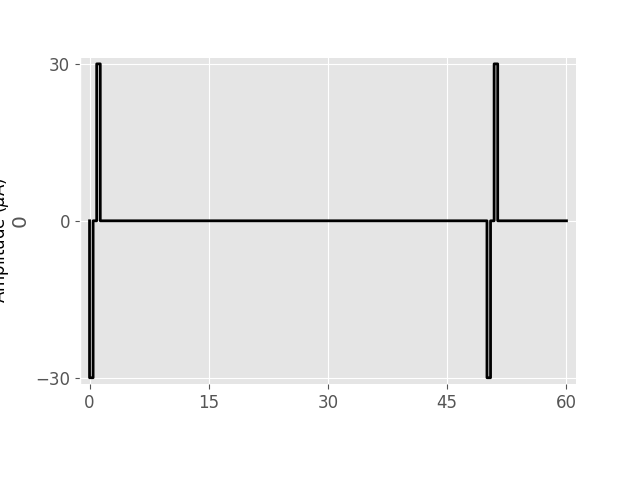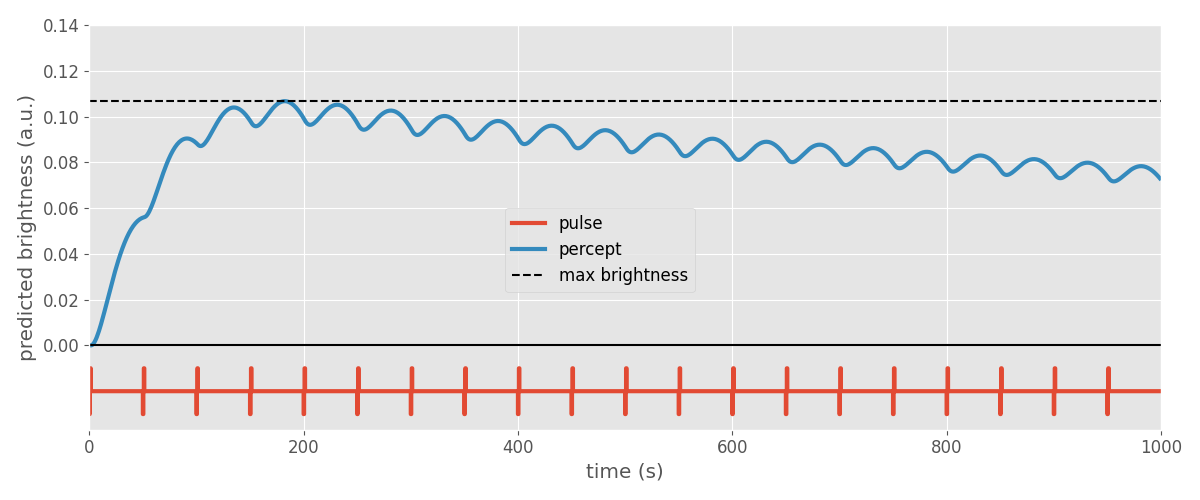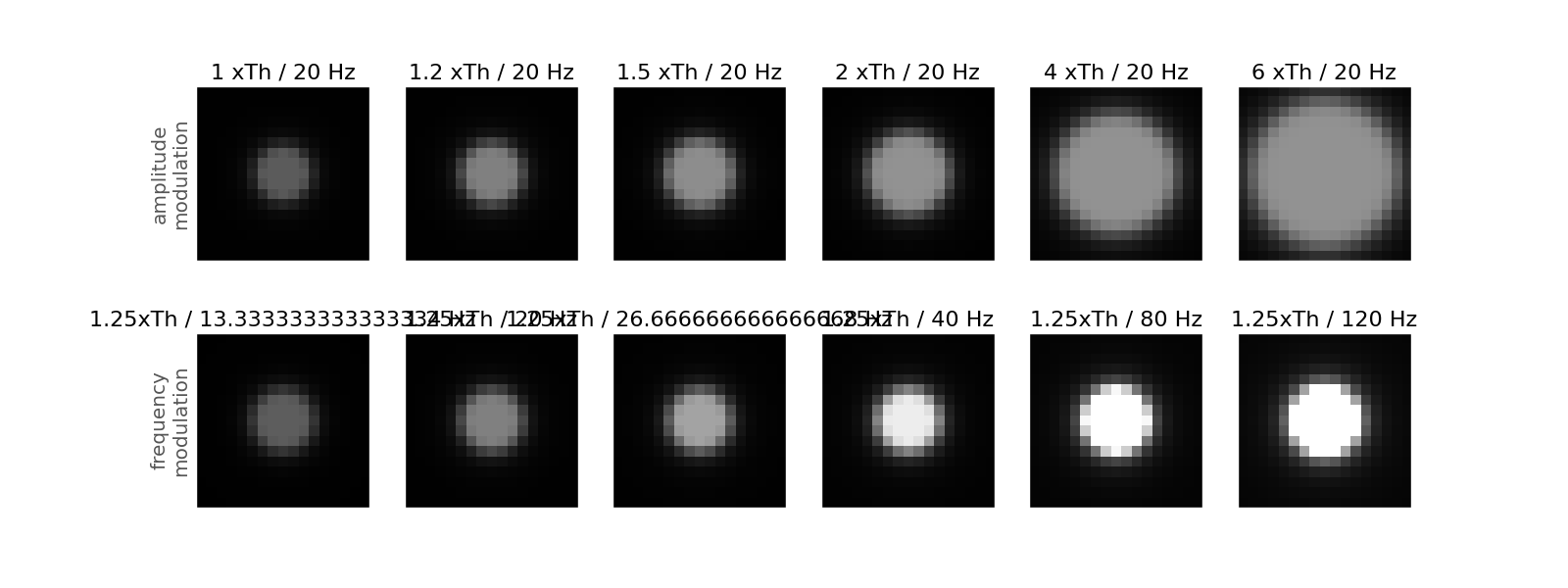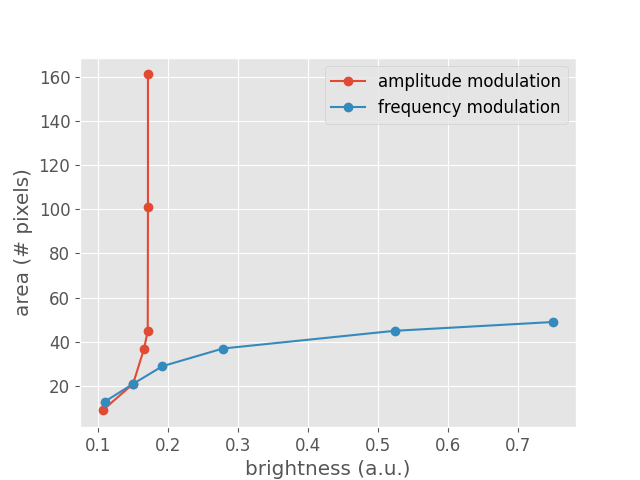# Nanduri et al. (2012): Frequency vs. amplitude modulation¶

This example shows how to use the Nanduri2012Model.

The model introduced in [Nanduri2012] assumes that electrical stimulation leads to percepts that quickly increase in brightness (over the time course of ~100ms) and then slowly fade away (over the time course of seconds). The model also assumes that amplitude and frequency modulation have different effects on the perceived brightness and size of a phosphene.

## Generating a pulse train¶

The first step is to build a pulse train using the PulseTrain class. We want to generate a 20Hz pulse train (0.45ms pulse duration, cathodic-first) at 30uA that lasts for a second:

from pulse2percept.stimuli import BiphasicPulseTrain, Stimulus
tsample = 0.005  # sampling time step (ms)
phase_dur = 0.45  # duration of the cathodic/anodic phase (ms)
stim_dur = 1000  # stimulus duration (ms)
amp_th = 30  # threshold current (uA)
stim = BiphasicPulseTrain(20, amp_th, phase_dur, interphase_dur=phase_dur,
stim_dur=stim_dur)

# Configure Matplotlib:
import matplotlib.pyplot as plt
plt.style.use('ggplot')
from matplotlib import rc
rc('font', size=12)

# Plot the stimulus in the range t=[0, 60] ms:
stim.plot(time=(0, 60))Out:

<AxesSubplot:ylabel='0'>


## Creating an implant¶

Before we can run the Nanduri model, we need to create a retinal implant to which we can assign the above pulse train.

For the purpose of this exercise, we will create an ElectrodeArray consisting of a single DiskElectrode with radius=260um centered at (x,y) = (0,0); i.e., centered over the fovea:

from pulse2percept.implants import DiskElectrode, ElectrodeArray
earray = ElectrodeArray(DiskElectrode(0, 0, 0, 260))


Usually we would use a predefined retinal implant such as ArgusII or AlphaIMS. Alternatively, we can wrap the electrode array created above with a ProsthesisSystem to create our own retinal implant. We will also assign the above created stimulus to it:

from pulse2percept.implants import ProsthesisSystem
implant = ProsthesisSystem(earray, stim=stim)


## Running the model¶

Interacting with a model always involves three steps:

1. Initalize the model by passing the desired parameters.
2. Build the model to perform (sometimes expensive) one-time setup computations.
3. Predict the percept for a given stimulus.

In the following, we will run the Nanduri model on a single pixel, (0, 0):

from pulse2percept.models import Nanduri2012Model
model = Nanduri2012Model(xrange=(0, 0), yrange=(0, 0))
model.build()


Out:

Nanduri2012Model(asymptote=14.0, atten_a=14000,
atten_n=1.69, dt=0.005, engine='serial',
eps=8.73, grid_type='rectangular',
n_jobs=1, scale_out=1.0,
slope=3.0, spatial=Nanduri2012Spatial,
tau1=0.42, tau2=45.25, tau3=26.25,
temporal=Nanduri2012Temporal,
thresh_percept=0, verbose=True,
xrange=(0, 0), xystep=0.25, yrange=(0, 0))


Note

You can also directly instantiate Nanduri2012Temporal and pass a stimulus to it. However, please note that there might be subtle differences; e.g., Nanduri2012Model will pass the stimulus through the spatial model first.

After building the model, we are ready to predict the percept.

The input to the model is an implant containing a stimulus (usually a pulse train), which is processed in time by a number of linear filtering steps as well as a stationary nonlinearity (a sigmoid).

import numpy as np
percept = model.predict_percept(implant, t_percept=np.arange(1000))


The output of the model is a Percept object that contains a time series with the predicted brightness of the visual percept at every time step.

“Brightness of a stimulus” is defined as the maximum brightness value encountered over time:

bright_th = percept.data.max()
bright_th


Out:

0.10671083


Plotting the percept next to the applied stimulus reveals that the model predicts the perceived brightness to increase rapidly (within ~100ms) and then drop off slowly (over the time course of seconds). This is consistent with behavioral reports from Argus II users.

fig, ax = plt.subplots(figsize=(12, 5))
ax.plot(implant.stim.time,
-0.02 + 0.01 * implant.stim.data[0, :] / implant.stim.data.max(),
linewidth=3, label='pulse')
ax.plot(percept.time, percept.data[0, 0, :], linewidth=3, label='percept')
ax.plot([0, stim_dur], [bright_th, bright_th], 'k--', label='max brightness')
ax.plot([0, stim_dur], [0, 0], 'k')

ax.set_xlabel('time (s)')
ax.set_ylabel('predicted brightness (a.u.)')
ax.set_yticks(np.arange(0, 0.14, 0.02))
ax.set_xlim(0, stim_dur)
fig.legend(loc='center')
fig.tight_layout()Note

In psychophysical experiments, brightness is usually expressed in relative terms; i.e., compared to a reference stimulus. In the model, brightness has arbitrary units.

## Brightness as a function of frequency/amplitude¶

The Nanduri paper reports that amplitude and frequency have different effects on the perceived brightness of a phosphene.

To study these effects, we will apply the model to a number of amplitudes and frequencies:

# Use the following pulse duration (ms):
pdur = 0.45
# Generate values in the range [0, 50] uA with a step size of 5 uA or smaller:
amps = np.linspace(0, 50, 11)
# Initialize an empty list that will contain the predicted brightness values:
bright_amp = []
for amp in amps:
# For each value in the amps vector, now stored as amp, do the
# following:
# 1. Generate a pulse train with amplitude amp, 20 Hz frequency, 0.5 s
#    duration, pulse duration pdur, and interphase gap pdur:
implant.stim = BiphasicPulseTrain(20, amp, pdur, interphase_dur=pdur,
stim_dur=stim_dur)
# 2. Run the temporal model:
percept = model.predict_percept(implant)
# 3. Find the largest value in percept, this will be the predicted
# brightness:
bright_pred = percept.data.max()
# 4. Append this value to bright_amp:
bright_amp.append(bright_pred)


We then repeat the procedure for a whole range of frequencies:

# Generate values in the range [0, 100] Hz with a step size of 10 Hz or
# smaller:
freqs = np.linspace(0, 100, 11)
# Initialize an empty list that will contain the predicted brightness values:
bright_freq = []
for freq in freqs:
# For each value in the amps vector, now stored as amp, do the
# following:
# 1. Generate a pulse train with amplitude amp, 20 Hz frequency, 0.5 s
#    duration, pulse duration pdur, and interphase gap pdur:
implant.stim = BiphasicPulseTrain(freq, 20, pdur, interphase_dur=pdur,
stim_dur=stim_dur)
# 2. Run the temporal model
percept = model.predict_percept(implant)
# 3. Find the largest value in percept, this will be the predicted
# brightness:
bright_pred = percept.data.max()
# 4. Append this value to bright_amp:
bright_freq.append(bright_pred)


Plotting the two curves side-by-side reveals that the model predicts brightness to saturate quickly with increasing amplitude, but to scale linearly with stimulus frequency:

fig, ax = plt.subplots(ncols=2, sharey=True, figsize=(12, 5))

ax.plot(amps, bright_amp, 'o-', linewidth=4)
ax.set_xlabel('amplitude (uA)')
ax.set_ylabel('predicted brightness (a.u.)')

ax.plot(freqs, bright_freq, 'o-', linewidth=4)
ax.set_xlabel('frequency (Hz)')

fig.tight_layout()## Phosphene size as a function of amplitude/frequency¶

The paper also reports that phosphene size is affected differently by amplitude vs. frequency modulation.

To introduce space into the model, we need to re-instantiate the model and this time provide a range of (x,y) values to simulate. These values are specified in degrees of visual angle (dva). They are sampled at xydva dva:

model = Nanduri2012Model(xystep=0.5, xrange=(-4, 4), yrange=(-4, 4))
model.build()


Out:

Nanduri2012Model(asymptote=14.0, atten_a=14000,
atten_n=1.69, dt=0.005, engine='serial',
eps=8.73, grid_type='rectangular',
n_jobs=1, scale_out=1.0,
slope=3.0, spatial=Nanduri2012Spatial,
tau1=0.42, tau2=45.25, tau3=26.25,
temporal=Nanduri2012Temporal,
thresh_percept=0, verbose=True,
xrange=(-4, 4), xystep=0.5,
yrange=(-4, 4))


We will again apply the model to a whole range of amplitude and frequency values taken directly from the paper:

# Use the amplitude values from the paper:
amp_factors = [1, 1.25, 1.5, 2, 4, 6]

# Output brightness in 1ms time steps:
t_percept = np.arange(0, stim_dur, 1)

# Initialize an empty list that will contain the brightest frames:
frames_amp = []

for amp_f in amp_factors:
# For each value in the amp_factors vector, now stored as amp_f, do:
# 1. Generate a pulse train with amplitude amp_f * amp_th, frequency
#    20Hz, 0.5s duration, pulse duration pdur, and interphase gap pdur:
implant.stim = BiphasicPulseTrain(20, amp_f * amp_th, pdur,
interphase_dur=pdur, stim_dur=stim_dur)
# 2. Run the temporal model:
percept = model.predict_percept(implant, t_percept=t_percept)
# 3. Save the brightest frame:
frames_amp.append(percept.get_brightest_frame())

# Use the amplitude values from the paper:
freqs = [40.0 / 3, 20, 2.0 * 40 / 3, 40, 80, 120]

# Initialize an empty list that will contain the brightest frames:
frames_freq = []

for freq in freqs:
# For each value in the freqs vector, now stored as freq, do:
# 1. Generate a pulse train with amplitude 1.25 * amp_th, frequency
#    freq, 0.5s duration, pulse duration pdur, and interphase gap
#    pdur:
implant.stim = BiphasicPulseTrain(freq, 1.25 * amp_th, pdur,
interphase_dur=pdur, stim_dur=stim_dur)
# 2. Run the temporal model:
percept = model.predict_percept(implant, t_percept=t_percept)
# 3. Save the brightest frame:
frames_freq.append(percept.get_brightest_frame())


This allows us to reproduce Fig. 7 of [Nanduri2012]:

fig, axes = plt.subplots(nrows=2, ncols=len(amp_factors), figsize=(16, 6))

for ax, amp, frame in zip(axes, amp_factors, frames_amp):
ax.imshow(frame, vmin=0, vmax=0.3, cmap='gray')
ax.set_title('%.2g xTh / 20 Hz' % amp, fontsize=16)
ax.set_xticks([])
ax.set_yticks([])
axes.set_ylabel('amplitude\nmodulation')

for ax, freq, frame in zip(axes, freqs, frames_freq):
ax.imshow(frame, vmin=0, vmax=0.3, cmap='gray')
ax.set_title('1.25xTh / %d Hz' % freq, fontsize=16)
ax.set_xticks([])
ax.set_yticks([])
axes.set_ylabel('frequency\nmodulation')Out:

Text(194.44444444444446, 0.5, 'frequency\nmodulation')


## Phosphene size as a function of brightness¶

Lastly, the above data can also be visualized as a function of brightness to highlight the difference between frequency and amplitude modulation (see Fig.8 of [Nanduri2012]):

plt.plot([np.max(frame) for frame in frames_amp],
[np.sum(frame >= bright_th) for frame in frames_amp],
'o-', label='amplitude modulation')
plt.plot([np.max(frame) for frame in frames_freq],
[np.sum(frame >= bright_th) for frame in frames_freq],
'o-', label='frequency modulation')
plt.xlabel('brightness (a.u.)')
plt.ylabel('area (# pixels)')
plt.legend()Out:

<matplotlib.legend.Legend object at 0x7f082ba77490>


Total running time of the script: ( 0 minutes 13.699 seconds)

Gallery generated by Sphinx-Gallery